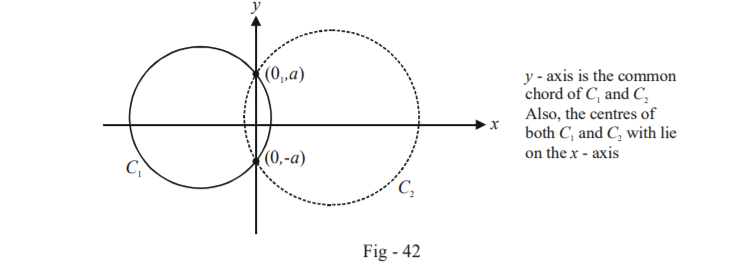# Examples On Common Chords And Radical Axes Set-2

Go back to  'Circles'

Example - 34

Prove that two circles, both of which pass through the point $$(0,a)$$ and $$(0, - a)$$ and touch the line $$y = mx + c,$$ will cut orthogonally if $${c^2} = {a^2}(2 + {m^2})$$

Solution: It should be clear that the $$y$$-axis is the common chord of the two circles $${C_1}$$ and $${C_2}$$ :Let the centres of $${C_1}$$ and $${C_2}$$ be  $$( - {g_1},0)$$ and $$( - {g_2},0)$$ so that their radii become $$\sqrt {g_1^2 + {a^2}}$$ and $$\sqrt {g_2^2 + {a^2}}$$ respectively. Their equations then become:

${C_1}:{x^2} + {y^2} + 2{g_1}x - {a^2} = 0$

${C_2}:{x^2} + {y^2} + 2{g_2}x - {a^2} = 0$

The line $$y = mx + c$$ touches both $${C_1}$$ and $${C_2}\,$$ so that the perpendicular distance of the centres of $${C_1}$$ and $${C_2}\,$$ from this line must be respectively equal to their radii. Thus, we obtain

\begin{align}&\frac{{\left| {m{g_1} - c} \right|}}{{\sqrt {1 + {m^2}} }} = \sqrt {g_1^2 + {a^2}}\;\text{and} \;\frac{{\left| {m{g_2} - c} \right|}}{{1 + {m^2}}} = \sqrt {g_2^2 + {a^2}} \\ \Rightarrow \qquad\;\; &g_1^2 + 2mc{g_1} + {a^2}(1 + {m^2}) - {c^2} = 0\\ \;\text{and} \qquad &g_2^2 + 2mc{g_2} + {a^2}(1 + {m^2}) - {c^2} = 0\end{align}

Thus, $${g_1}$$ and $${g_2}$$ are the roots of the equation

${g^2} + 2mcg + {a^2}(1 + {m^2}) - {c^2} = 0$

so that

${g_1}{g_2} = {a^2}(1 + {m^2}) - {c^2}$

Finally, $${C_1}$$ and $${C_2}$$ are orthogonal if the condition

$2({g_1}{g_2} + {f_1}{f_2}) = {c_1} + {c_2}$

is satisfied, i.e.

\begin{align} & 2\{ ({a^2}(1 + {m^2}) - {c^2}) + (0)(0)\} = - 2{a^2}\\ \Rightarrow \qquad & {c^2} = {a^2}(2 + {m^2}) \end{align}

This is the required relation for  and $${C_2}$$ to be orthogonal.

Example - 35

Consider the following two circles:

\begin{align} &{S_1}:{x^2} + {y^2} - 16 = 0\\ &{S_2}:{x^2} + {y^2} - 8x - 12y + 16 = 0\end{align}

Circles are drawn which are orthogonal to both these circles. Tangents are drawn from the centre of the variable circle to $${S_1}$$. Find the mid locus of the mid-point of the chord of contact so formed.

Solution: The centre of the variable circle, say $$({x_1},{y_1}),$$ lies on the radical axis of the two given circles, i.e. on $${S_1} - {S_2} = 0$$ by the result obtained in Example - 33.

Thus, $$({x_1},{y_1})$$ must satisfy

\begin{align} & {S_1} - {S_2} = 0\;{\rm{or}}\;8x + 12y - 32 = 0\\ \Rightarrow \qquad & 8{x_1} + 12{y_1} - 32 = 0 & & ...(1) \end{align}

From $$({x_1},{y_1}),$$ two tangents are drawn to $${S_1}.$$ The equation of the chord of contact is therefore

\begin{align} &T({x_1},{y_1}) = 0\\ \Rightarrow \qquad & x{x_1} + y{y_1} = 16 \qquad\qquad\qquad ...(2) \end{align}

Let the mid-point of the chord of contact so formed be $$M(h,k).$$ We can also write the equation of the same chord of contact using the equation we derived for a chord bisected at a given point.

Thus, the chord of contact can also be represented by the equation

\begin{align}& T(h,k) = S(h,k)\\\Rightarrow \qquad & hx + ky = {h^2} + {k^2} \qquad \qquad ...(3)\end{align}

Since (2) and (3) are the same lines, we have

\begin{align} & \frac{{{x_1}}}{h} = \frac{{{y_1}}}{k} = \frac{{16}}{{{h^2} + {k^2}}}\\ \Rightarrow \qquad & {x_1} = \frac{{16h}}{{{h^2} + {k^2}}};\,\,{y_1} = \frac{{16k}}{{{h^2} + {k^2}}}\qquad \qquad \dots \rm{(4)}\end{align}

Using (4) in (1), we finally obtain a relation in $$(h,k)$$:

\begin{align} & 8{x_1} + 12{y_1} - 32 = 0\\ \Rightarrow \qquad & \frac{{128h}}{{{h^2} + {k^2}}} + \frac{{192k}}{{{h^2} + {k^2}}} = 32\\\Rightarrow \qquad &{h^2} + {k^2} - 4h - 6k = 0\end{align}

Using $$(x,y)$$ instead of $$(h,k)$$ we obtain the required locus as

${x^2} + {y^2} - 4x - 6y = 0$

## TRY YOURSELF - III

Q. 1 Find the equation of the circle which intersects $${x^2} + {y^2} - 6x + 4y - 3 = 0$$  orthogonally, passes through $$(3, 0)$$ and touches the $$y$$-axis.

Q. 2 At what angle do the circles

\begin{align}{S_1}:{x^2} + {y^2} - 4x + 6y + 11 = 0\\{S_2}:{x^2} + {y^2} - 2x + 8y + 13 = 0\end{align}

intersect?

Q. 3 Find the co-ordinates of the point from which the tangents drawn to the following three circles are of equal lengths:

\begin{align} & {S_1}:3{x^2} + 3{y^2} + 4x - 6y - 1 = 0\\ & {S_2}:2{x^2} + 2{y^2} - 3x - 2y - 4 = 0\\ &{S_3}:2{x^2} + 2{y^2} - x + y - 1 = 0\end{align}

Q. 4 Find the locus of the centre of the circle passing through $$(a,b)$$ and orthogonal to $${x^2} + {y^2} = {k^2}.$$

Q. 5 Let $$A({a_1},{b_1})\,$$ and $$B({a_2},{b_2})$$ be two fixed points and $$O$$ be the origin. Circles are drawn on OA and OB as diameters. Prove that the length of the common chord is \begin{align}\frac{{\left| {{a_1}{b_2} - {a_2}{b_1}} \right|}}{{AB}}\end{align} .

Circles
grade 11 | Questions Set 1
Circles
Circles
grade 11 | Questions Set 2
Circles